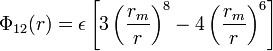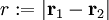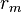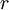# 8-6 Lennard-Jones potential

Jump to: navigation, search

The 8-6 Lennard-Jones potential (also known as the 6-8 potential) is a variant the more well known Lennard-Jones model. It is particularly useful for computing non-bonded interactions. The potential is given by (Eq. 4 in):$\Phi_{12}(r) = \epsilon \left[ 3\left(\frac{r_m}{r} \right)^{8} - 4\left( \frac{r_m}{r}\right)^6 \right]$

where

•$r := |\mathbf{r}_1 - \mathbf{r}_2|$
•$\Phi_{12}(r)$ is the intermolecular pair potential between two particles or sites
•$r_m$ is the distance,$r$, at which$\Phi_{12}(r)$ is a minimum.
•$\epsilon$ is the well depth (energy)